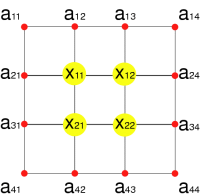Fall 2003

# Mathematics Math21b Fall 2003

## Linear Algebra and Differential Equations

Office: SciCtr 434
Email: knill@math.harvard.edu# The Tension of a Drum

(An example, where a huge system of equations appear in mechanics)
 As an example of a system with many variables, consider a drum modeled by a fine net. The heights at each interior node needs the average the heights of the 4 neighboring nodes. The height at the boundary is fixed. With n2 nodes in the interior, we have to solve a system A x=b of n2 equations. The problem solved here is a discrete version of the Dirichlet problem, which we will see later in class and solve explicitly.``` x11=a21+a12+x21+x12 x12=x11+x13+x22+x22 x21=x31+x11+x22+a43 x22=x12+x21+a43+a34 ```

 Fixing the heights at the boundary and the requirement that inside, the heights are the averages of the neighboring heights fixes the heights everywhere.Numerical solution of a situation with n=24. The computer was asked to solve the system of linear equations.

 The problem has other interpretations. If we had given the temperature distribution at the boundary, then the solution would give the temperature distribution inside the region. We could replace temperature also with voltage and replace each connection between nodes by a resistor. The solution would give then the voltage distribution inside the resistor network.Numerical solution with n=200. The computer had to solve a system of 40'000 unknowns.

Back to the main page